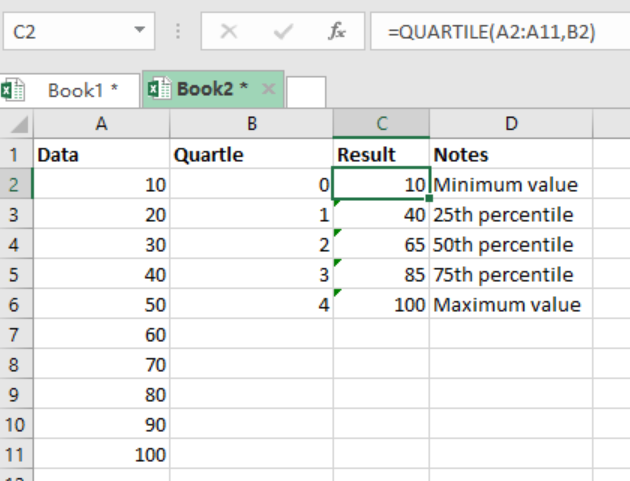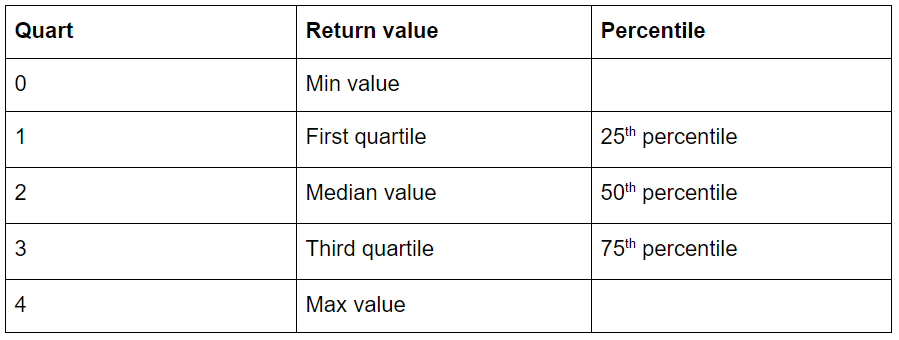Get instant live expert help with Excel or Google Sheets“My Excelchat expert helped me in less than 20 minutes, saving me what would have been 5 hours of work!”

#### Post your problem and you’ll get Expert help in seconds.

Your message must be at least 40 characters
Our professional Expert are available now. Your privacy is guaranteed.

# Excel QUARTILE Function

If we want to get the quartile of a given set of data, we can use the Excel QUARTILE function. We can get the minimum value, first, second, third quartiles and the maximum value. To understand how to find the quartiles of a given set of data, read this post to the end.Figure 1: Finding quartiles in excel

## Syntax of the formula

`=QUARTILE (array, quart)`

Where;

Array- reference with data to analyze

Quart- quartile value be to returned

## Explanation

When we want to get the quartiles of a set of data, we use the Excel QUARTILE function. This function takes two arguments, the array and the quart. The array contains the numeric data which we want to analyze while the quart indicates the quartile value to return. The function only accepts 5 values for the quart. This can be seen from the dropdown list when you are entering the formula.

In our example above, the formula in cell C2 is

`=QUARTILE(A2:A11,B2)`

We then copy the formula down across the other cells to find the other quartiles.

The table below shows the 5 return values accepted by this function## Instant Connection to an Expert through our Excelchat Service

Most of the time, the problem you will need to solve will be more complex than a simple application of a formula or function. If you want to save hours of research and frustration, try our live Excelchat service! Our Excel Experts are available 24/7 to answer any Excel question you may have. We guarantee a connection within 30 seconds and a customized solution within 20 minutes.

### Did this post not answer your question? Get a solution from connecting with the expert.Another blog reader asked this question today on Excelchat:## Subscribe to Excelchat.coAnother blog reader asked this question today on Excelchat: